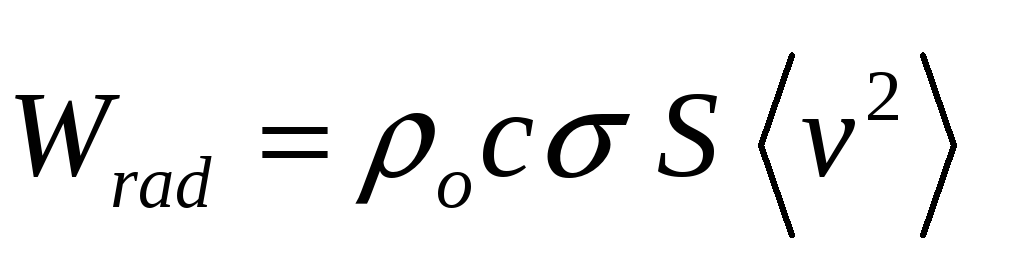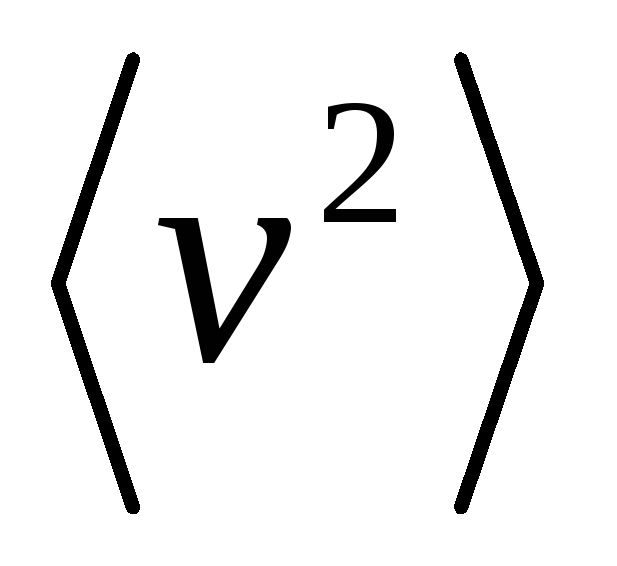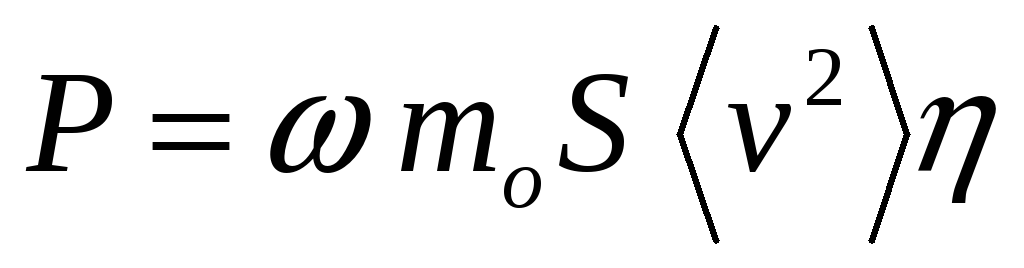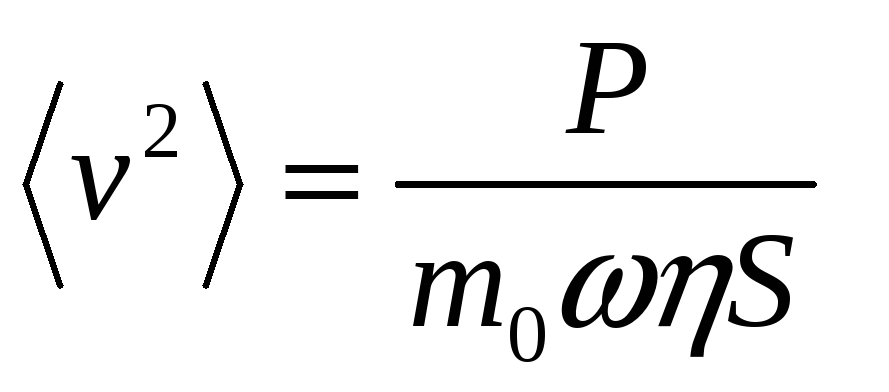# Measurement of structure borne power

 Page 2/3 Date 31.03.2018 Size 229.61 Kb.
1   2   3

## Measurement of structure borne power

Given the inherent installation dependence of structure-borne sound sources, it is clearly difficult to develop a suitable standard for measuring it.
One existing standard relates to equipment for the water supply industry, ISO 3822 , where a water pipe is connected to a wall, and noise radiation from the wall is measured. There is no direct measure of the power produced by the test item, instead a comparison is made with a reference source of noise in the water pipe, an orifice, and the noise of the test item is ranked relative to that. Whilst this does provide useful information to the industry, a recent publication suggests that some of the assumptions in this standard do not apply  and that that this leads to problems in rank-ordering appliances.
The method of ISO3822 is superficially similar to the reception plate method described in reference  although the latter does provide a measurement of the power flowing into the receiver plate. One conclusion to note, however, is that “It remains to be considered how the reception plate power, obtained with the machine under test in the laboratory, can be transformed to yield the installed power from the same machine in a building”. The answer to this problem lies either in a suitable scaling law for the mobility of the building compared with the reception plate, or possibly in the concept of characteristic power introduced by Moorhouse .
A final method of characterising machinery is through ISO9611 , which specifies the measurement of velocity of contact points of isolated machinery. Combining the velocity data with information about the stiffness of the isolators can be used to infer a first order estimate of the forces at the mounting points.

1. ## Prediction methods for noise

The power flowing into the building structure is mainly a nuisance because of the re-radiated noise, and so it is useful to extend the power flow modelling to consider that aspect. For radiation from the main wall the radiated sound power is given from(3)
Where ρ0c is the characteristic impedance of air, σ is the radiation efficiency of the wall, S is surface area andis the space averaged mean square velocity. The energy balance equation for the plate gives that(4)

and so(5)
It is clear that increasing the mass of the wall, whilst keeping stiffness constant, reduces radiated sound power both indirectly by reducing the mechanical power input through equation (2), and directly by reducing the mean square velocity through equation (5). An additional small benefit is that radiation efficiency may be reduced at low frequencies as adding mass tends to increase the coincidence frequency.
Predictions where there is more than one wall, as in figure 1, require more sophisticated models such as finite element analysis (FEA) at low frequencies or statistical energy analysis (SEA) at higher frequencies. The prediction model of EN12354 uses what is essentially a simplified SEA model, with examples of its application being found in references  and , both papers showing reasonable agreement with measured data.

1   2   3